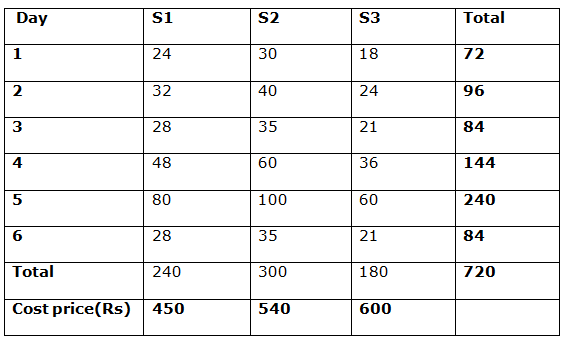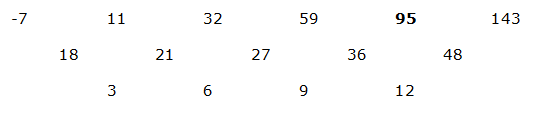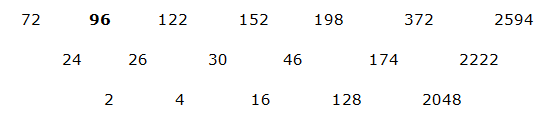# IBPS Clerk Mains Quantitative Aptitude Questions 2019 (Day-13) High Level New Pattern

Dear Aspirants, Quantitative Aptitude plays a crucial role in Banking and all other competitive exams. To enrich your preparation, here we have provided New Pattern Aptitude Questions for IBPS Clerk Mains. Candidates those who are going to appear in IBPS Clerk Mains can practice these questions daily and make your preparation effective.

[WpProQuiz 4827]

Directions (1 -5): Read the following information carefully and answer the given questions.

Shivani, a saree seller bought 720 sarees of 3 different types (S1, S2 and S3) in the ratio 4: 5: 3. She sells the sarees based on market demand. On the 1st day he sells 10% of the total volume. On the 2nd day she sells (40/3) % of the total volume. On the 3rd day she sells (35/3) % of the total volume. On the 4th day she sells 20% of the total volume. On the 5th day she sells 33(1/3) % of the total volume. On the 6th day she sells the same volume as on the 3rd day.

The cost of each S1, S2 and S3 sarees is Rs. 450, Rs. 540 and Rs. 600 respectively. On each day she sells the sarees of each variety in the same ratio as she bought.

1) If on the 3rd day she sells S3 saree for a price which is more than 20% than their cost price whereas the other two sarees are sold at their cost price, then find the total amount received by Shivani by selling the sarees on the 3rd day?

a) Rs. 42540

b) Rs. 48750

c) Rs. 46620

d) Rs. 49620

e) Rs. 44570

2) If on the 4th day she marks up 60% for all the three type of sarees and allows 20%, 15% and 18% discount on S1, S2 and S3 type sarees respectively. Find the total amount received by Shivani by selling the sarees on the 4th day?

a) Rs. 105001.25

b) Rs. 105010.2

c) Rs. 150000.2

d) Rs. 120500.2

e) None of these

3) Total S1 type sarees sold on the 2nd, 3rd and 4th day is what percentage more/less than the total S3 type sarees sold on the 1st, 5th and 6th day?

a) 9.09%

b) 18.08%

c) 18.18%

d) 22.22%

e) 11.11%

4) In which of the following day the amount received by Shivani is maximum if all the sarees sold the same as cost price?

a) 2nd day

b) 5th day

c) 3rd day (or) 6th day

d) 4th day

e) 1st day

5) It is known that 25% of the sarees were slightly damaged in each day. If Shivani managed to sell the sarees which were slightly damaged at 20% loss, then find the amount made by Shivani for selling the damaged sarees on the 2nd, 4th and 5th day. (Consider the ratio of the damaged sarees in the three categories is same as the ratio which she bought)?

a) Rs. 42500

b) Rs. 36800

c) Rs. 72540

d) Rs. 50400

e) Rs. 84680

Directions (6 -10): A number is bolded in the series I, II and III. Find the wrong series which does not satisfies the given number and choose the options accordingly.

6)

I. 12, 96, 672, 4032, 20160, 80640

II. -7, 11, 32, 59, 96, 143

III. 72, 96, 122, 152, 198, 372, 2594

a) Only III

b) Only II

c) Only I

d) Both I and II

e) Both II and III

7)

I. 79, 200, 369, 594, 883, 1244

II. 1365, 1509, 1313, 1569, 1244, 1645

III. 3171, 3046, 2830, 2487, 1975, 1244

a) Both I and II

b) Only I

c) Only III

d) Both II and III

e) None

8)

I. 24, 25, 54, 159, 640, 3205

II. 12, 26, 54, 110, 222, 446

III. 12, 13, 28, 29, 54, 61, 124, 125

a) Only II

b) Only III

c) Both I and III

d) Only I

e) None

9)

I. 64, 33, 34, 52, 105, 264

II. 32, 16, 24, 60, 212, 945

III. 128, 65, 68, 141, 571, 4576

a) None

b) Only I

c) Only III

d) Only II

e) All the three

10)

I. 9, 19, 59, 239, 1199, 7200

II. 78, 156, 468, 2340, 16380

III. 72, 77, 87, 102, 122, 145

a) Both II and III

b) Only I

c) Only III

d) Both I and III

e) All the three

Directions (1 -5):

Total sarees = 720

Ratio of S1, S2 and S3 is 4: 5: 3

S1 type sarees = 720 * 4/12 = 240

S2 type sarees = 720 * 5/12 = 300

S3 type sarees = 720 * 3/12 = 180

On the 1st day:

Total sarees sold = 720 * 10/100 = 72

Ratio of S1, S2 and S3 is 4: 5: 3

S1 type sarees = 72 * 4/12 = 24

S2 type sarees = 72 * 5/12 = 30

S3 type sarees = 72 * 3/12 = 18

On the 2nd day:

Total sarees sold = 720 * 40/300 = 96

Ratio of S1, S2 and S3 is 4: 5: 3

S1 type sarees = 96 * 4/12 = 32

S2 type sarees = 96 * 5/12 = 40

S3 type sarees = 96 * 3/12 = 24

On the 3rd day:

Total sarees sold = 720 * 35/300 = 84

Ratio of S1, S2 and S3 is 4: 5: 3

S1 type sarees = 84 * 4/12 = 28

S2 type sarees = 84 * 5/12 = 35

S3 type sarees = 84 * 3/12 = 21

On the 4th day:

Total sarees sold = 720 * 20/100 = 144

Ratio of S1, S2 and S3 is 4: 5: 3

S1 type sarees = 144 * 4/12 = 48

S2 type sarees = 144 * 5/12 = 60

S3 type sarees = 144 * 3/12 = 36

On the 5th day:

Total sarees sold = 720 * 100/300 = 240

Ratio of S1, S2 and S3 is 4: 5: 3

S1 type sarees = 240 * 4/12 = 80

S2 type sarees = 240 * 5/12 = 100

S3 type sarees = 240 * 3/12 = 60

On the 6th day:

Total sarees sold = 720 * 35/300 = 84

Ratio of S1, S2 and S3 is 4: 5: 3

S1 type sarees = 84 * 4/12 = 28

S2 type sarees = 84 * 5/12 = 35

S3 type sarees = 84 * 3/12 = 21The cost of each S1, S2 and S3 saree is Rs.450, Rs. 540 and Rs.600 respectively

The selling price of each S1, S2 and S3 saree is,

SP of S1 = CP of S1 = 450

SP of S2 = CP of S2 = 540

SP of S3 = 120% of CP of S3 = (120/100)*600 = 720

Total amount received by Shivani = (28 *450) + (35*540) + (21*720)

= 12600 + 18900 + 15120

= Rs. 46620

MP of S1 type sarees = 450 * 160/100 = 720

SP of S1 type sarees = 720 * 80/100 = 576

MP of S2 type sarees = 540 * 160/100 = 864

SP of S2 type sarees = 864 * 85/100 = 734.4

MP of S3 type sarees = 600 * 160/100 = 960

SP of S3 type sarees = 960 * 82/100 = 787.2

= (48*576) + (60*734.4) + (36*787.2)

= 27648 + 44064 + 28339.2

= Rs. 100051.2

Total S1 type sarees sold on 2nd, 3rd and 4th day

= 32 + 28 + 48 = 108

Total S3 type sarees sold on the 1st, 5th and 6th day

= 18 + 60 + 21 = 99

Required percentage = [(108 – 99)/99] *100 = 9.09%

On the 1st day,

Total amount = (24*450) + (30*540) + (18*600)

= 10800 + 16200 + 10800

= 37800

On the 2nd day,

Total amount = (32*450) + (40*540) + (24*600)

= 14400 + 21600 + 14400

= 50400

On the 3rd day,

Total amount = (28*450) + (35*540) + (21*600)

= 12600 + 18900 + 12600

= 44100

On the 4th day,

Total amount = (48*450) + (60*540) + (36*600)

= 21600 + 32400 + 21600

= 75600

On the 5th day,

Total amount = (80*450) + (100*540) + (60*600)

= 36000 + 54000 + 36000

= 126000

On the 6th day,

Total amount = (28*450) + (35*540) + (21*600)

= 12600 + 18900 + 12600

= 44100

On 5th day, Shivani received the maximum amount.

(Or)

In which day she sold maximum sarees on that day she received maximum amount.

From the above information, we could find 5th day she received maximum amount.

SP of S1 type sarees = 450 *80/100 = 360

SP of S2 type sarees = 540 * 80/100 = 432

SP of S3 type sarees = 600 * 80/100 = 480

On the 2nd day:

Total damaged sarees sold = 96 * 25/100 = 24

Ratio of S1, S2 and S3 is 4: 5: 3

S1 type sarees = 24 * 4/12 = 8

S2 type sarees = 24 * 5/12 = 10

S3 type sarees = 24 * 3/12 = 6

= (8*360) + (10*432) + (6*480)

= 2880 + 4320 + 2880

= Rs. 10080

On the 4th day:

Total damaged sarees sold = 144 * 25/100 = 36

Ratio of S1, S2 and S3 is 4: 5: 3

S1 type sarees = 36 * 4/12 = 12

S2 type sarees = 36 * 5/12 = 15

S3 type sarees = 36 * 3/12 = 9

= (12*360) + (15*432) + (9*480)

= 4320 + 6480 + 4320

= Rs. 15120

On the 5th day:

Total damaged sarees sold = 240 * 25/100 = 60

Ratio of S1, S2 and S3 is 4: 5: 3

S1 type sarees = 60 * 4/12 = 20

S2 type sarees = 60 * 5/12 = 25

S3 type sarees = 60 * 3/12 = 15

= (20*360) + (25*432) + (15*480)

= 7200 + 10800 + 7200

= 25200

Total amount = 10080 + 15120 + 25200 = Rs. 50400

Direction (6-10) :

Series – I pattern:

12 * 8 = 96

96 * 7 = 672

672 * 6 = 4032

4032 * 5 = 20160

20160 * 4 = 80640

The bolded number is followed the given series.

Series – II pattern:The bolded number is not followed the given series.

Series – III pattern:The bolded number is followed the given series.

Series – I pattern:

79 + 121 (112) = 200

200 + 169 (132) = 369

369 + 225 (152) = 594

594 + 289 (172) = 883

883 + 361 (192) = 1244

The bolded number is followed the given series.

Series – II pattern:

1365 + 144 (122) = 1509

1509 – 196 (142) = 1313

1313 + 256 (162) = 1569

1569 – 324 (182) = 1245

1245 + 400 (202) = 1645

The bolded number is not followed the given series.

Series – III pattern:

3171 – 125 (53) = 3046

3046 – 216 (63) = 2830

2830 – 343 (73) = 2487

2487 – 512 (83) = 1975

1975 – 729 (93) = 1246

The bolded number is not followed the given series.

Series – I pattern:

24 * 1 + 1 = 25

25 * 2 + 2 = 52

52 * 3 + 3 = 159

159 * 4 + 4 = 640

640 * 5 + 5 = 3205

The bolded number is not followed the given series.

Series – II pattern:

12 * 2 + 2 = 26

26 *2 + 2 = 54

54 * 2 + 2 = 110

110 * 2 + 2 = 222

222 * 2 + 2 = 446

The bolded number is followed the given series.

Series – III pattern:

12 * 1 + 1 = 13

13 * 2 + 2 = 28

28 * 1 + 1 = 29

29 * 2 + 2 = 60

60 * 1 + 1 = 61

61 * 2 + 2 = 124

124 * 1 + 1 = 125

The bolded number is not followed the given series.

Series – I pattern:

64 * 0.5 + 1 = 33

33 * 1 + 1 = 34

34 * 1.5 + 1 = 52

52 * 2 + 1 = 105

105 * 2.5 + 1 = 263.5

The bolded number is not followed the given series.

Series – II pattern:

32 * 0.5 = 16

16 * 1.5 = 24

24 * 2.5 = 60

60 * 3.5 = 210

210 * 4.5 = 945

The bolded number is not followed the given series.

Series – III pattern:

128 * 0.5 + 1 = 65

65 * 1 + 3 = 68

68 * 2 + 5 = 141

141 * 4 + 7 = 571

571 * 8 + 9 = 4577

The bolded number is not followed the given series.

Series – I pattern:

9 * 2 + 1 = 19

19 * 3 + 2 = 59

59 * 4 + 3 = 239

239 * 5 + 4 = 1199

1199 * 6 + 5 = 7199

The bolded number is not followed the given series.

Series – II pattern:

78*2 = 156

156*3 = 468

468 * 5 = 2340

2340 * 7 = 16380

The bolded number is followed the given series.

Series – III pattern:

72 + 5 = 77

77 + 10 = 87

87 + 15 = 102

102 + 20 = 122

122 + 25 = 147

The bolded number is not followed the given series.